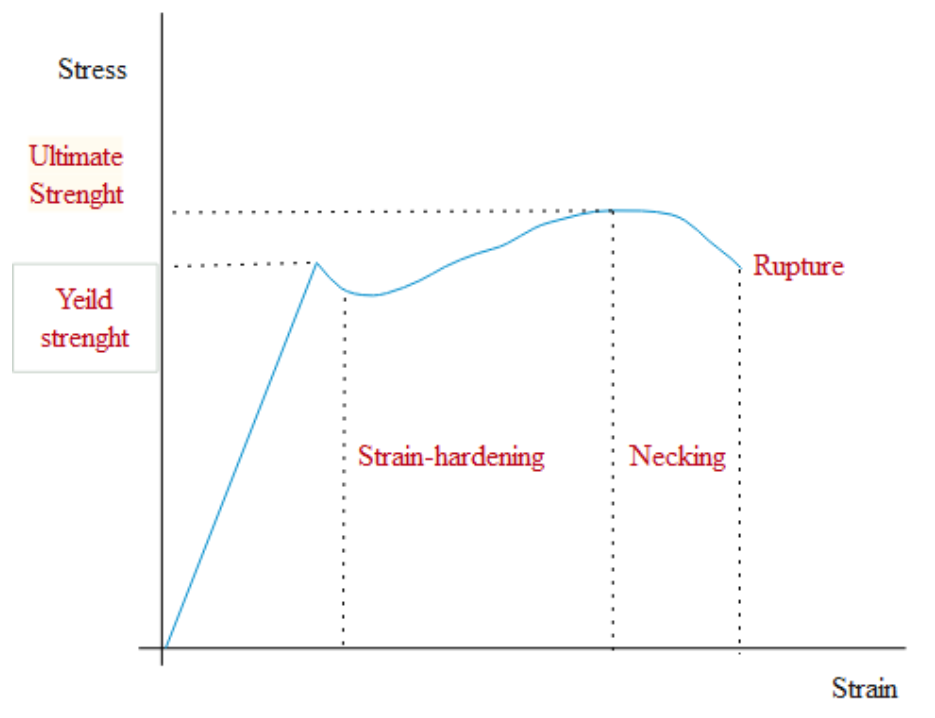Courses
Courses for Kids
Free study material
Free LIVE classes
More# Hooke's law states that under normal conditions:A. Stress is inversely proportional to strain till the elastic limitB. Stress is directly proportional to strain till the elastic limitC. Stress is independent of strainD. Stress is proportional to the elastic modulusVerified
288.3k+ views
Hint: According to Hooke's law the force required to move a mass attached to a spring whose one end is fixed at a point is equal to the product of distance moved by the body and the constant factor of the spring. This was given by the physicist Robert Hooke in the $17^{th}$ – century. This law is used to study the deformation of anybody.

Complete step-by-step solution:
The force required to move a mass attached to a spring whose one end is fixed at a point is equal to the product of distance moved by the body and the constant factor of the spring. This statement was given by and published by Robert Hooke in his anagram in 1678. And therefore the statement is termed as Hooke’s Law.
$F=-kx$
The stresses and strains of material inside a constant elastic material (for example a block of rubber, steel bar) are connected by a linear relationship that is mathematically the same as Hooke's law.
The deforming force can be applied to a material by stretching, compressing, squeezing, bending, or twisting. So, a metal wire exhibits elastic behavior because according to Hooke’s law the small increase in its length when stretched by an applied force doubles each time the force is doubled.Hooke’s law is only applicable to the elastic properties of a material when it is in the range when force and displacement are proportional. This can be expressed in the terms of stress and strain.
According to Hooke's law within the elastic limit, the stress is directly proportional to the strain on the body.
Thus, the correct option is Option B.

Note: Elasticity of a material represents the ability of the material to restore its original state. When an external deformable force is applied the body gets deformed. Once the force is removed the body should regain its original state with its properties of elasticity.
Last updated date: 25th May 2023
Total views: 288.3k
Views today: 6.46k